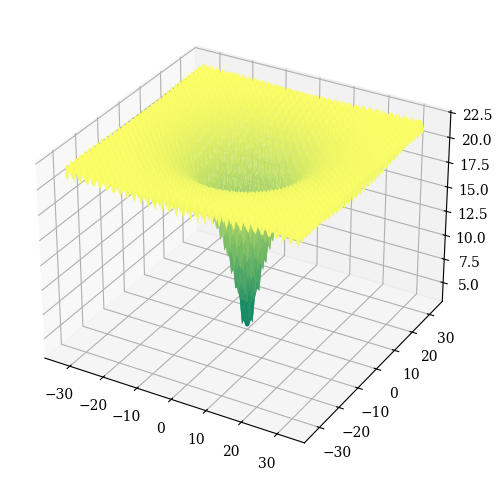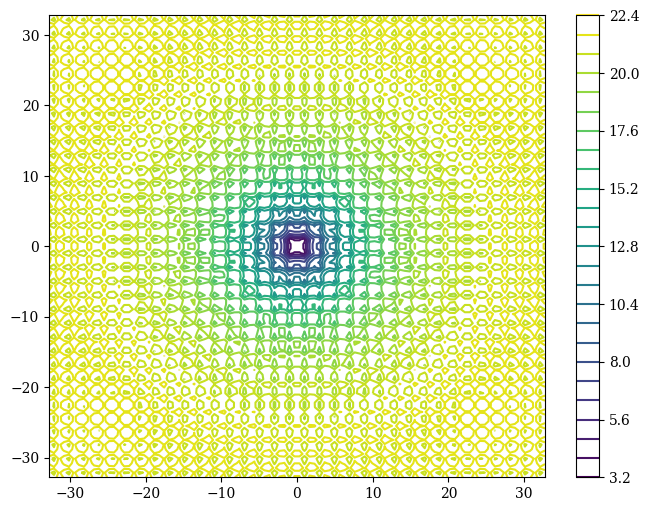Version: 0.6.0

# Ackley¶

The Ackley function is widely used for testing optimization algorithms. In its two-dimensional form, as shown in the plot above, it is characterized by a nearly flat outer region, and a large hole at the centre. The function poses a risk for optimization algorithms, particularly hillclimbing algorithms, to be trapped in one of its many local minima.

Definition

\begin{align} \begin{split} f(x) &=& \,-a \exp{ \Bigg[ -b \, \sqrt{ \frac{1}{n} \sum_{i=1}^{n}{x_i}^2 } \Bigg]} - \exp{ \Bigg[ \frac{1}{n}\sum_{i=1}^{n}{cos(c x_i)} \Bigg] } + a + e, \2mm] && a = \;20, \quad b = \; \frac{1}{5}, \quad c = \;2 \pi \\[2mm] &&-32.768 \leq x_i \leq 32.768, \quad i=1 \ldots,n \\[4mm] \end{split} \end{align} Optimum \[f(x^*) = 0 \; \text{at} \; x^* = (0,\ldots,0)

Fitness Landscape

:

import numpy as np

from pymoo.problems import get_problem
from pymoo.visualization.fitness_landscape import FitnessLandscape

problem = get_problem("ackley", n_var=2, a=20, b=1/5, c=2 * np.pi)

FitnessLandscape(problem, angle=(45, 45), _type="surface").show()

:

<pymoo.visualization.fitness_landscape.FitnessLandscape at 0x7f886997ea90>:

FitnessLandscape(problem, _type="contour", colorbar=True).show()

:

<pymoo.visualization.fitness_landscape.FitnessLandscape at 0x7f8836ea6e50>